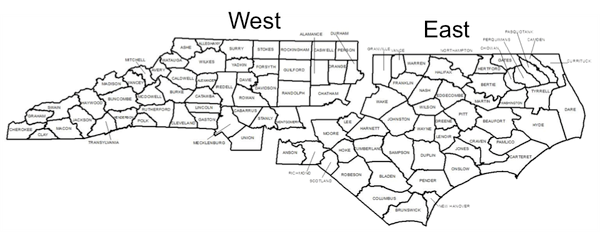NC State Extension Publications

## Introduction

Hardwood lumber’s nature as a producer good piques interests in its price across the forest value chain. Secondary manufacturers are keenly aware of their raw material costs. The margin present between kiln-dried and green lumber prices can provide insights into the economics of the drying process.

The prices received by sawmillers represent the fixed and variable costs incurred to convert standing hardwood sawtimber into lumber, along with some provisions for profits and risk along each step of the process. Five year trends can provide forest landowners an understanding of the hardwood production cycle when considering a timber sale.

The prices presented in this note over the previous five years were compiled from Weekly Hardwood Review (WHR), which is distributed by Hardwood Publishing of Charlotte, NC. WHR provides market prices for a number of individual species or species groups across the eastern U.S. and Canada. Prices are provided for both green/air-dried (Gr/AD) and kiln-dried (KD) lumber for the following appearance grades- First and Seconds combined with First and Seconds 1 Face (FAS/1F), #1 Common (#1C), and #2 Common (#2C). Specifications for meeting each grade conform to National Hardwood Lumber Association rules (or slight variations).

Gr/AD prices for #3 common lumber are also listed in limited cases. Plus, prices are given for a number of non-grade hardwood products. While the quality of these non-grade products is not necessarily predicated on their appearance, some may indeed be evaluated for strength and durability. A detailed discussion of hardwood lumber pricing can be found in Luppold (1996).

## North Carolina Hardwood Lumber Markets

North Carolina is split into two lumber market regions by WHR. The delineation between the state’s western and eastern lumber markets closely follows the timber market regions outlined in NC State Extension Forestry’s compilation of historic timber prices (Bardon 2016a, 2016b). Western North Carolina is part of WHR’s southern Appalachian market region. Eastern North Carolina is within WHR’s Southern market region.Figure 1. North Carolina lumber market regions (Source: Bardon 2016a, 2016b; map created by Jessica Knight).

## North Carolina Hardwood Lumber Prices

Nominal prices paid at the time for Gr/AD and KD lumber by grade are illustrated in the tables below. The prices are aggregations across a mix of key commercial species present in each region, weighted by their relative contributions to the respective region’s sawtimber inventory in 2010. They have not been adjusted for inflation. Quarterly averages (as determined by the geometric mean) were developed from WHR’s market reports. Per WHR, prices are quoted FOB mill in full truckload or carload quantities (U.S. dollars per thousand board feet, $/MBF). Prices for western North Carolina are comprised of four groups of species- Soft (Red) Maple (Acer rubrum), Select and Non-select Red Oaks (Quercus spp.), Select and Non-select White Oaks (Quercus spp.), and Yellow-poplar (Liriodendron tulipifera). Prices for eastern North Carolina are comprised of three species groups- Select and Non-select Red Oaks, Select and Non-select White Oaks, and Yellow-poplar. An example of a select red oak in western North Carolina is northern red oak (Quercus rubra) while black oak (Quercus velutina) would be considered non-select. In eastern North Carolina cherrybark oak (Quercus pagoda) is a select species while southern red oak (Quercus falcata) is non-select. White oak (Quercus alba) is a select species in both regions. Taken together, these steps serve three purposes. One, the proprietary nature of WHR’s market data is protected. Second, the aggregated prices can be thought of as representing a typical mix of appearance grade lumber produced by a hardwood sawmill in each region. Third, reporting lumber prices on a quarterly basis allows direct comparisons to be made with NC State Extension Forestry’s timber price reports for mixed hardwood sawtimber.  Year- Quarter FAS/1F #1C #2C 2011-Q1$757 $480$369 2011-Q2 $777$472 $364 2011-Q3$773 $479$362 2011-Q4 $766$478 $356 2012-Q1$803 $487$355 2012-Q2 $853$506 $362 2012-Q3$853 $508$373 2012-Q4 $875$524 $405 2013-Q1$917 $564$443 2013-Q2 $959$595 $492 2013-Q3$985 $635$507 2013-Q4 $1,026$650 $532 2014-Q1$1,121 $700$550 2014-Q2 $1,169$768 $565 2014-Q3$1,143 $781$560 2014-Q4 $1,117$764 $550 2015-Q1$1,111 $744$526 2015-Q2 $1,047$668 $457 2015-Q3$988 $582$420 2015-Q4 $1,013$572 $412  Year-Quarter FAS/1F #1C #2C 2011-Q1$1,109 $775$626 2011-Q2 $1,129$764 $615 2011-Q3$1,118 $772$614 2011-Q4 $1,088$736 $583 2012-Q1$1,101 $721$569 2012-Q2 $1,149$746 $573 2012-Q3$1,162 $760$586 2012-Q4 $1,168$760 $593 2013-Q1$1,197 $786$603 2013-Q2 $1,257$829 $645 2013-Q3$1,300 $887$696 2013-Q4 $1,345$928 $748 2014-Q1$1,441 $1,012$820 2014-Q2 $1,513$1,099 $863 2014-Q3$1,520 $1,133$878 2014-Q4 $1,483$1,104 $862 2015-Q1$1,466 $1,059$817 2015-Q2 $1,429$983 $723 2015-Q3$1,386 $881$653 2015-Q4 $1,373$835 $623  Year Quarter FAS/1F #1C #2C 2011-Q1$709 $453$368 2011-Q2 $720$458 $364 2011-Q3$715 $451$357 2011-Q4 $689$436 $343 2012-Q1$711 $446$330 2012-Q2 $771$475 $341 2012-Q3$783 $481$352 2012-Q4 $796$502 $393 2013-Q1$837 $557$442 2013-Q2 $880$586 $476 2013-Q3$913 $599$493 2013-Q4 $954$628 $519 2014-Q1$1,052 $698$559 2014-Q2 $1,101$733 $570 2014-Q3$1,079 $735$566 2014-Q4 $1,045$712 $550 2015-Q1$1,039 $683$523 2015-Q2 $989$602 $454 2015-Q3$934 $527$420 2015-Q4 $950$536 $421  Year Quarter FAS/1F #1C #2C 2011-Q1$1,001 $696$598 2011-Q2 $1,022$699 $596 2011-Q3$987 $694$571 2011-Q4 $950$655 $527 2012-Q1$969 $656$526 2012-Q2 $1,035$683 $549 2012-Q3$1,043 $693$558 2012-Q4 $1,061$698 $567 2013-Q1$1,111 $740$608 2013-Q2 $1,192$817 $662 2013-Q3$1,243 $858$706 2013-Q4 $1,283$919 $771 2014-Q1$1,379 $1,019$863 2014-Q2 $1,467$1,110 $896 2014-Q3$1,469 $1,108$898 2014-Q4 $1,422$1,058 $857 2015-Q1$1,404 $1,002$767 2015-Q2 $1,366$900 $684 2015-Q3$1,303 $802$622 2015-Q4 $1,272$788 \$603

## References

Bardon, R. 2016a. Historic North Carolina timber stumpage prices, 1976-2015. N.C. Cooperative Extension note.

Bardon, R. 2016b. Historic North Carolina delivered timber prices, 1988-2015. N.C. Cooperative Extension note.

Luppold, W. 1996. An explanation of hardwood lumber pricing. Forest Products Journal 46(5):52-55.

Weekly Hardwood Review. 2011 to 2015. Weekly market reports. Hardwood Publishing, Charlotte, NC.

# Author

Extension Specialist, Forest Biomaterials
Wood Products Extension